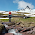## 28/06/2013

### [SQL] Matrix multiplication

The second assignment in my Coursera Big Data course regarded data analysis in SQL using SQLite3. We used a test dataset, which you can download from here, composed of:
•  matrix.db: a simple database representing two square sparse matrices stored as tables A and B each with row_num, col_num and value as columns
• reuters.db: a database containing a single table frequency(docid, term, count), where docid is an identifier corresponding to a particular file, term is an English word, and count is the number of the times the term appears within the document indicated by docid

I will not post all scripts produced here since many were just simple SELECTs so we'll just focus on the most interesting stuff: matrix multiplication in SQL.

It sounds more complicated than it really is but, given the two sparse matrices, it is possible to compute their multiplication AxB by doing a simple JOIN between A columns and B rows, then GROUPing BY A rows and B columns and finally SELECTing, for each matrix cell, the SUM of the multiplication between the two cell values in both matrices:

SELECT a.row_num, b.col_num, SUM(a.value*b.value)
FROM A a JOIN B b ON a.col_num=b.row_num
GROUP BY a.row_num, b.col_num;

Which is exactly the implementation of the matrix multiplication formula.

If you're interested in the other tasks for this assignment, I'll post below the instructions along the solution scripts. They must all be run against the reuters DB.

NOTE: Since I cannot use Greek characters, I'll write [SIGMA] and [PI] to indicate SELECT and PROJECT in relational algebra.

(a) select: Write a query that is equivalent to the following relational algebra expression: Solution

[SIGMA]docid=10398_txt_earn(frequency)

(b) select project: Write a SQL statement that is equivalent to the following relational algebra expression: Solution

[PI]term([SIGMA]docid=10398_txt_earn and count=1(frequency))

(c) union: Write a SQL statement that is equivalent to the following relational algebra expression: Solution

[PI]term([SIGMA]docid=10398_txt_earn and count=1(frequency)) U [PI]term( [SIGMA]docid=925_txt_trade and count=1(frequency))

(d) count: Write a SQL statement to count the number of documents containing the word “parliament”. Solution

(e) big documents: Write a SQL statement to find all documents that have more than 300 total terms, including duplicate terms. Solution

(f) two words: Write a SQL statement to count the number of unique documents that contain both the word 'transactions' and the word 'world'. Solution

(g) assignment g was to compute the AxB matrix multiplication described above.

(h) similarity matrix: Write a query to compute the similarity matrix DDT (transposed matrix). The query could take some time to run if you compute the entire result. Solution

(i) keyword search: Find the best matching document to the keyword query "washington taxes treasury". Solution

#### 2 comments:

1.That's really helpful! Could you please explain how the transpose works?
The solution is there, but if you could walk through it, that would be great.

1.Hi,

the transpose is trivial enough as it's just a swap of the rows and columns of the matrix. You could do it as:

CREATE VIEW transpose (row, column, value)
AS SELECT column, row, value FROM matrix;

The example H however, shows how to compute the similarity matrix, which is the multiplication of the matrix D with its transpose DT. The reuters dataset provided is a term-document matrix where each row is a document vector, with one column for every term in the entire corpus; since some documents may not contain a given term, the matrix is sparse. The value in each cell of the matrix is the term frequency. When computing the similarity for the sample set provided, if two documents both contain a term, then the score goes up by the product of the two term frequencies. This score is equivalent to the dot product of the two document vectors.

Furthermore, instead of computing the full solution, the assignment asked to find only the similarity of the two documents '10080_txt_crude' and '17035_txt_earn', that's the reason for the WHERE clause. Lastly, by joining column on column instead of column on row, you get the create the transponse within the query.

With great power comes great responsibility.

Da grandi poteri derivano grandi responsabilità.